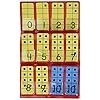hand2mind DecaDots Ten Frame Math Tiles for Kids, Ten Frame Math Manipulatives, Counting Toys for Counting, Place Value…

Amazon.com Price: \$16.18 (as of 15/01/2022 05:57 PST- Details)

Make sure this fits by entering your model number.
MATH SKILLS DEVELOPMENT: DecaDots Ten Frame tiles provide a more intuitive and visual representation of patterns for numbers up to 10, than traditional ten frame tiles. DecaDots Ten Frame Tiles help students learn subtilizing and understand the crucial concept of making 5, 10, and 20. Children learn to recognize the number of objects in a group without having to count the objects.
TEN FRAME MATH: Ten frame tiles allow kids to visualize early math concepts such as counting, addition, subtraction, number patterns, making 10, number relationships, and number correspondence. Kids quickly learn shortcuts such as counting the spaces remaining (counting back from 10) instead of counting the number of dots.hand2mind DecaDots Ten Frame Math Tiles for Kids, Ten Frame Math Manipulatives, Counting Toys for Counting, Place Value…

Amazon.com Price: \$16.18 (as of 15/01/2022 05:57 PST- Details)

Category: